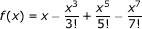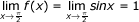# Calculus - Calculus MCQ

16:

If x + y = k, x > 0, y > 0, then xy is maximum when

 A. x = ky B. kx = y C. x = y D. None of these Answer Report Discuss Option: C Explanation : Click on Discuss to view users comments. Write your comments here:
17:

Which of the following is correct ?

 A. f(a) is an extreme value of f(x) if f '(a)=0 B. If f(a) is an extreme value of f(x), then f '(a) = 0 C. If f '(a) = 0, then f (a) is an extreme value of f(x) D. all of these Answer Report Discuss Option: D Explanation : Click on Discuss to view users comments. Write your comments here:
18:

Let f(x) = lxl, then

 A. f'(0) = 0 B. f(x) is maximum at x = 0 C. f(x) is minimum at x = 0 D. None of these Answer Report Discuss Option: D Explanation : Click on Discuss to view users comments. Write your comments here:
19:

The maxima and minima of the function
f(x) = 2x3 - 15x2 + 36x + 10 occur respectively at

 A. x= 3 and x = 2 B. x = 1 and x = 3 C. x = 2 and x = 3 D. x = 3 and x = 4 Answer Report Discuss Option: C Explanation : f(x)=2x3-15x2+36x+10 f '(x)=6x2-30x+36 and f ''(x)=12x-30 For maxima and minima, f '(x)=0  6x2-30x+36 = 0 or x2- 5x + 6 = 0 or  X = 3,2 Putting these values of x in equation (ii), we get f '(3) = 36 - 30 = + 6 positive Hence minimum value = 3 f ' (2) = 24 - 30 = - 6 negative, hence maximum value = 2 Click on Discuss to view users comments. Write your comments here:
20:

Limit of the following series as x approaches π/2 isA. 2π/3 B. π/2 C. π/3 D. 1 Answer Report Discuss Option: D Explanation :Click on Discuss to view users comments. Write your comments here: